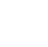Back to Chapter
C++ Setting UpC++ IntroductionNo items found.
C++ BasicsNo items found.
C++ Control StatementsNo items found.
C++ Logical OperatorsNo items found.
C++ Procedural ProgrammingNo items found.
C++ Structural ProgrammingNo items found.
C++ Implementation of OOPSNo items found.
C++ Arrays and VectorsNo items found.
C++ Error HandlingNo items found.
C++ File HandlingNo items found.

# C++ Vectors and Dynamic Arrays

### Dynamic Arrays

Dynamic memory allocation in C/C++ refers to performing memory allocation manually by programmer.

One use of dynamically allocated memory is to allocate memory of variable size which is not possible with compiler allocated memory.

The most important use is flexibility provided to programmers. We are free to allocate and deallocate memory whenever we need and whenever we don’t need anymore. There are many cases where this flexibility helps.

For normal variables like `int a`, `char str', etc, memory is automatically allocated and deallocated. For dynamically allocated memory like int *A = new int, it is the programmer's responsibility to deallocate memory when no longer needed. If the programmer doesn’t deallocate memory, it causes a memory leak.

C offers  malloc() and calloc() functions to allocate memory dynamically at run time and uses free() function to free dynamically allocated memory. C++ supports these functions while also offering two operators new and delete that perform the task of allocating and freeing the memory in a better and easier way.

C++ code to demonstrate the use of new and delete

``` #include using namespace std; int main () { // Pointer initialization to null int* A = NULL; // Request memory for the variable // using new operator A = new(nothrow) int; if (!A) cout << "allocation of memory failed\n"; else { // Store value at allocated address *A = 29; cout << "Value of A: " << *A<< endl; } // Request block of memory // using new operator float *R = new float(75.25); cout << "Value of R: " << *R<< endl; // Request block of memory of size n int n = 5; int *Q = new(nothrow) int[n]; if (!Q) cout << "allocation of memory failed\n"; else { for (int i = 0; i < n; i++) Q[i] = i+1; cout << "Value store in block of memory: "; for (int i = 0; i < n; i++) cout << Q[i] << " "; } // freed the allocated memory delete A; delete R; // freed the block of allocated memory delete[] Q; return 0; } ```
``` Output: Value of p: 29 Value of r: 75.25 Value store in block of memory: 1 2 3 4 5 ```

### Vectors

Vectors are the same as dynamic arrays with the ability to change themselves automatically when an element is inserted or deleted, with their storage being handled automatically by the container. Vector elements are placed in contiguous storage so that they can be accessed and traversed using iterators. In vectors, data is inserted at the end.

Certain functions associated with the vector are

1. begin()– Returns an iterator pointing to the first element in the vector
2. end()– Returns an iterator pointing to the theoretical element that follows the last element in the vector
3. rbegin()– Returns a reverse iterator pointing to the last element in the vector (reverse beginning). It moves from last to the first element
4. rend() – Returns a reverse iterator pointing to the theoretical element preceding the first element in the vector (considered as reverse end)
5. cbegin() – Returns a constant iterator pointing to the first element in the vector.
6. cend() – Returns a constant iterator pointing to the theoretical element that follows the last element in the vector.
7. crbegin()– Returns a constant reverse iterator pointing to the last element in the vector (reverse beginning). It moves from last to the first element
8. crend()– Returns a constant reverse iterator pointing to the theoretical element preceding the first element in the vector (considered as reverse end)

C++ program to demonstrate vectors in C++

``` #include #include using namespace std; int main() { vector V1; for (int i = 1; i <= 5; i++) V1.push_back(i); cout << "Output of begin and end: "; for (auto i = V1.begin(); i != V1.end(); ++i) cout << *i << " "; /*The auto keyword specifies that the type of the variable that is being declared will be automatically deducted from its initializer.*/ cout << "\nOutput of cbegin and cend: "; for (auto i = V1.cbegin(); i != V1.cend(); ++i) cout << *i << " "; cout << "\nOutput of rbegin and rend: "; for (auto ir = V1.rbegin(); ir != V1.rend(); ++ir) cout << *ir << " "; cout << "\nOutput of crbegin and crend : "; for (auto ir = V1.crbegin(); ir != V1.crend(); ++ir) cout << *ir << " "; return 0; } ```
``` Output: Output of begin and end: 1 2 3 4 5 Output of cbegin and cend: 1 2 3 4 5 Output of rbegin and rend: 5 4 3 2 1 Output of crbegin and crend : 5 4 3 2 1 ```